# c++：-7

2022-05-13 16:03 2221 查看

##范型程序设计

• 编写不依赖于具体数据类型的程序
• 将算法从特定的数据结构中抽象出来，成为通用的
• C++的模板为泛型程序设计奠定了关键的基础 ###概念 （1）用来界定具备一定功能的数据类型。例如：
• 将“可以比大小的所有数据类型（有比较运算符）”这一概念记为Comparable
• 将“具有公有的复制构造函数并可以用‘=’赋值的数据类型”这一概念记为Assignable
• 将“可以比大小、具有公有的复制构造函数并可以用‘=’赋值的所有数据类型”这个概念记作Sortable

（2）对于两个不同的概念A和B，如果概念A所需求的所有功能也是概念B所需求的功能，那么就说概念B是概念A的子概念。例如：

• Sortable既是Comparable的子概念，也是Assignable的子概念 ###模型

• int型是Comparable概念的模型。
• 静态数组类型不是Assignable概念的模型（无法用“=”给整个静态数组赋值） ####用概念做模板参数名
• 很多STL的实现代码就是使用概念来命名模板参数的。
• 为概念赋予一个名称，并使用该名称作为模板参数名。
• 例如：表示insertionSort这样一个函数模板的原型：
```template <class Sortable>
void insertionSort(Sortable a[], int n);```

##STL

• 标准模板库（Standard Template Library，简称STL）定义了一套概念体系，为泛型程序设计提供了逻辑基础
• STL中的各个类模板、函数模板的参数都是用这个体系中的概念来规定的。
• 使用STL的模板时，类型参数既可以是C++标准库中已有的类型，也可以是自定义的类型——只要这些类型是所要求概念的模型。

###介绍 ####STL的基本组件

• 容器（container）
• 迭代器（iterator）
• 函数对象（function object）
• 算法（algorithms）

####STL的基本组件间的关系 （1）* Iterators（迭代器）是算法和容器的桥梁。

• 将迭代器作为算法的参数、通过迭代器来访问容器而不是把容器直接作为算法的参数。

（2）将函数对象作为算法的参数而不是将函数所执行的运算作为算法的一部分。 （3）使用STL中提供的或自定义的迭代器和函数对象，配合STL的算法，可以组合出各种各样的功能。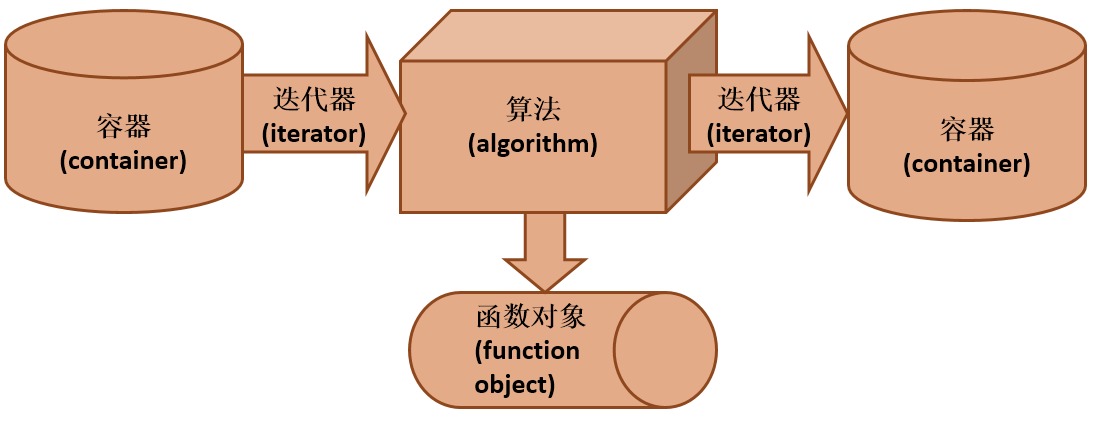###容器（container）

• 容器类是容纳、包含一组元素或元素集合的对象。
• 基于容器中元素的组织方式：顺序容器、关联容器
• 按照与容器所关联的迭代器类型划分：可逆容器随机访问容器

####容器分类

• unorderedset （无序集合）、unorderedmultiset（无序多重集合）
• unorderedmap（无序映射）、unordermultimap（无序多重映射）
• set（集合）、multiset（多重集合）、map（映射）、multimap（多重映射）
• array（数组）、vector（向量）、deque（双端队列）、forward_list（单链表）、list（列表）
• 顺序容器
• (有序)关联容器
• 无序关联容器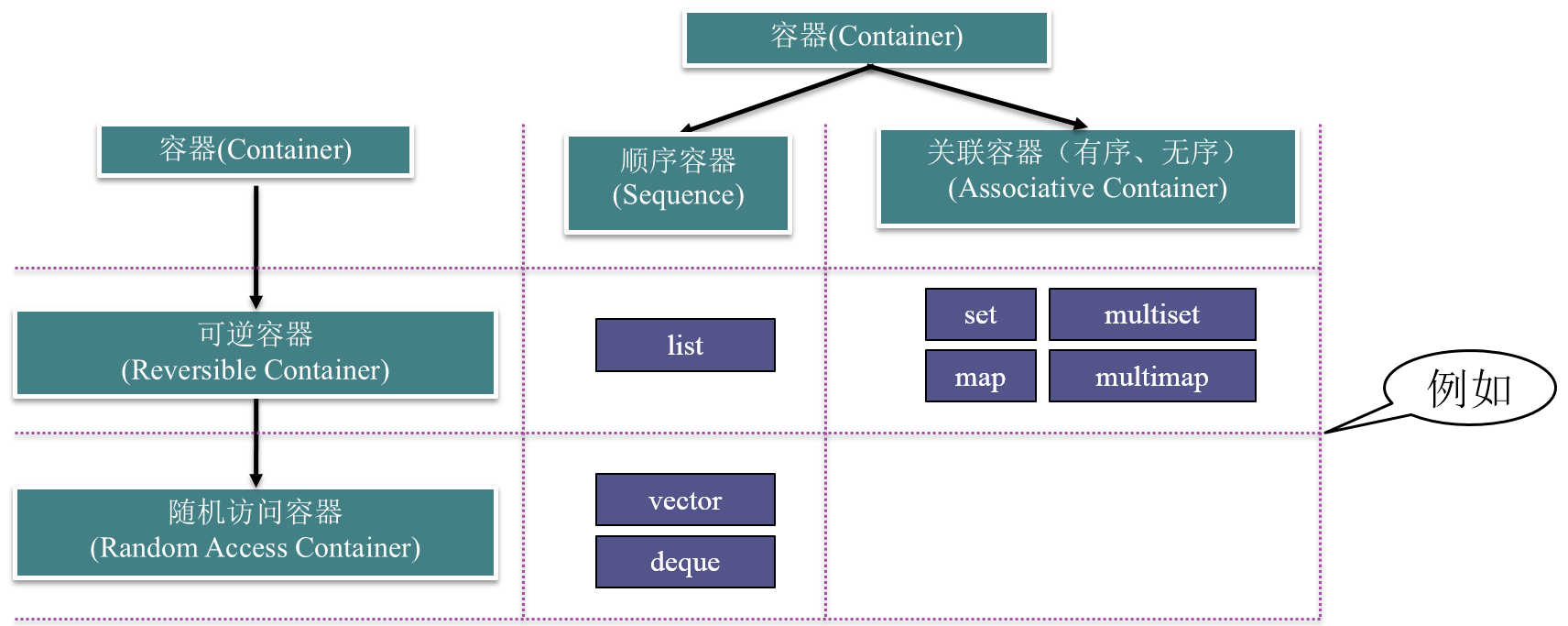####容器适配器

• stack（栈）、queue（队列）、priority_queue（优先队列）

####通用功能

• 用默认构造函数构造空容器
• 支持关系运算符：==、!=、<、<=、>、>=
• begin()、end()：获得容器首、尾迭代器
• clear()：将容器清空
• empty()：判断容器是否为空
• size()：得到容器元素个数
• s1.swap(s2)：将s1和s2两容器内容交换

####对可逆容器的访问 （1）STL为每个可逆容器都提供了逆向迭代器，逆向迭代器可以通过下面的成员函数得到：

• rbegin() ：指向容器尾的逆向迭代器
• rend()：指向容器首的逆向迭代器 （2）逆向迭代器的类型名的表示方式如下：
• S::reverse_iterator：逆向迭代器类型
• S::constreverseiterator：逆向常迭代器类型

####随机访问容器 随机访问容器支持对容器的元素进行随机访问 s
：获得容器s的第n个元素

###迭代器（iterator）

• 迭代器是泛化的指针，提供了顺序访问容器中每个元素的方法
• 提供了顺序访问容器中每个元素的方法；
• 可以使用“++”运算符来获得指向下一个元素的迭代器；
• 可以使用“*”运算符访问一个迭代器所指向的元素，如果元素类型是类或结构体，还可以使用“->”运算符直接访问该元素的一个成员；
• 有些迭代器还支持通过“--”运算符获得指向上一个元素的迭代器；
• 迭代器是泛化的指针：指针也具有同样的特性，因此指针本身就是一种迭代器；
• 使用独立于STL容器的迭代器，需要包含头文件

###函数对象（function object）

• 一个行为类似函数的对象，对它可以像调用函数一样调用。
• 函数对象是泛化的函数：任何普通的函数和任何重载了“()” 运算符的类的对象都可以作为函数对象使用
• 使用STL的函数对象，需要包含头文件

###算法（algorithms）

• STL包括70多个算法 例如：排序算法，消除算法，计数算法，比较算法，变换算法，置换算法和容器管理等
• 可以广泛用于不同的对象和内置的数据类型。
• 使用STL的算法，需要包含头文件 ####举例

```#include "iostream"
#include "vector"
#include "iterator"
#include "algorithm"
#include "functional"

using namespace std;
int main()
{
const int N=5;
vector<int> s(N);
for(int i=0;i<N;i++)
{
cin >> s[i];
}
transform(s.begin(),s.end(),ostream_iterator<int>(cout," "),negate<int>());
cout <<endl;
return 0;
}```

transform算法的一种实现：

```template <class InputIterator, class OutputIterator, class UnaryFunction>
OutputIterator transform(InputIterator first, InputIterator last, OutputIterator result, UnaryFunction op) {
for (;first != last; ++first, ++result)
*result = op(*first);
return result;
}```
• transform算法顺序遍历first和last两个迭代器所指向的元素；
• 将每个元素的值作为函数对象op的参数；
• 将op的返回值通过迭代器result顺序输出；
• 遍历完成后result迭代器指向的是输出的最后一个元素的下一个位置，transform会将该迭代器返回 ##迭代器 （1）迭代器是算法和容器的桥梁
• 迭代器用于访问容器中的元素
• 算法不直接操作容器中的数据，而是通过迭代器间接操作

（2）算法和容器独立

• 增加新的算法，无需影响容器的实现
• 增加新的容器，原有的算法也能适用

###输入输出迭代器 ####输入流迭代器

`istream_iterator<T>`
• 以输入流（如cin）为参数构造
• 可用*(p++)获得下一个输入的元素

####输出流迭代器

`ostream_iterator<T>`
• 构造时需要提供输出流（如cout）
• 可用(*p++) = x将x输出到输出流

• 适配器是用来为已有对象提供新的接口的对象
• 输入流适配器和输出流适配器为流对象提供了迭代器的接口

###分类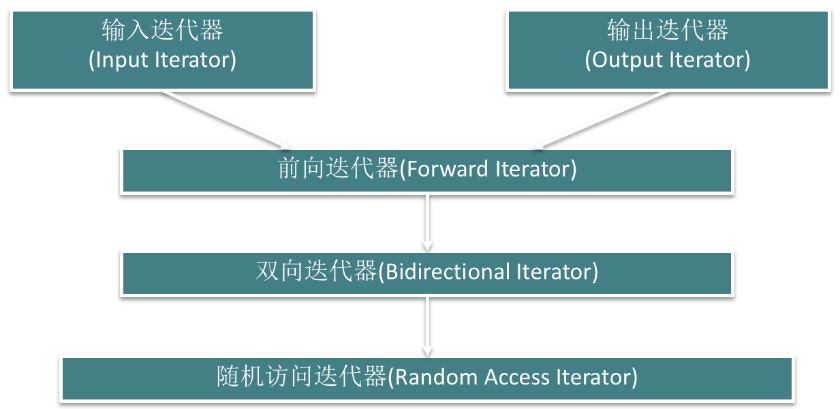（1）输入迭代器 可以用来从序列中读取数据，如输入流迭代器 （2）输出迭代器 允许向序列中写入数据，如输出流迭代器 （3）前向迭代器 既是输入迭代器又是输出迭代器，并且可以对序列进行单向的遍历 （4）双向迭代器 与前向迭代器相似，但是在两个方向上都可以对数据遍历 （5）随机访问迭代器 也是双向迭代器，但能够在序列中的任意两个位置之间进行跳转，如指针、使用vector的begin()、end()函数得到的迭代 ###迭代器的区间 （1）两个迭代器表示一个区间：[p1, p2) （2）STL算法常以迭代器的区间作为输入，传递输入数据 （3）合法的区间 p1经过n次(n > 0)自增(++)操作后满足p1 == p2 （4）区间包含p1，但不包含p2

###举例 （1）从标准输入读入几个实数，分别将它们的平方输出

```#include <iterator>
#include <iostream>
#include <algorithm>
using namespace std;

//求平方的函数
double square(double x) {
return x * x;
}
int main() {
//从标准输入读入若干个实数，分别将它们的平方输出
transform(istream_iterator<double>(cin), istream_iterator<double>(),
ostream_iterator<double>(cout, "\t"), square);
cout << endl;
return 0;
}

1 2 3 4
1	4	9	16```

（2） 综合运用几种迭代器的示例：程序涉及到输入迭代器、输出迭代器、随机访问迭代器这三个迭代器概念，并且以前两个概念为基础编写了一个通用算法。

```#include <algorithm>
#include <iterator>
#include <vector>
#include <iostream>
using namespace std;

//模版迭代器
//将来自输入迭代器的n个T类型的数值排序，将结果通过输出迭代器result输出
template <class T, class InputIterator, class OutputIterator>
void mySort(InputIterator first, InputIterator last, OutputIterator result) {
//通过输入迭代器将输入数据存入向量容器s中
vector<T> s;
for (;first != last; ++first)
{
s.push_back(*first);//对s进行排序，sort函数的参数必须是随机访问迭代器
}
sort(s.begin(), s.end());
copy(s.begin(), s.end(), result);   //将s序列通过输出迭代器输出
}

int main() {
//将s数组的内容排序后输出
double a = { 1.2, 2.4, 0.8, 3.3, 3.2 };
mySort<double>(a, a + 5, ostream_iterator<double>(cout, " "));
cout << endl;
//从标准输入读入若干个整数，将排序后的结果输出
mySort<int>(istream_iterator<int>(cin), istream_iterator<int>(), ostream_iterator<int>(cout, " "));
cout << endl;
return 0;
}```

##顺序容器 包括：

• 向量（vector）
• 双端队列（deque）
• 列表（list）
• 单向链表（forward_list）

###基本功能 （1）特点

• 元素线性排列，可以随时在指定位置插入元素和删除元素。
• 必须符合Assignable这一概念（即具有公有的拷贝构造函数并可以用“=”赋值）。
• array对象的大小固定，forward_list有特殊的添加和删除操作。

（2）接口（不包含单向链表（forward_list）和数组（array））

• 构造函数
• 赋值函数：assign
• 插入函数：insert， pushfront（只对list和deque）， pushback，emplace，emplace_front
• 删除函数：erase，clear，popfront（只对list和deque） ，popback，emplace_back
• 首尾元素的直接访问：front，back
• 改变大小：resize

（3）举例

```#include <iostream>
#include <list>
#include <deque>
using namespace std;

//输出指定的顺序容器的元素
template <class T>
void printContainer(const char* msg, const T& s) {
cout << msg << ": ";
copy(s.begin(), s.end(), ostream_iterator<int>(cout, " "));//s.begin()指向s的第一个元素（头），s.end()指向s的最后一个元素（尾）的下一个
cout << endl;
}

int main() {
//从标准输入读入10个整数，将它们分别从s的头部加入（头）
deque<int> s;
for (int i = 0; i < 10; i++) {
int x;
cin >> x;
s.push_front(x);
}
printContainer("deque at first", s);
//用s容器的内容的逆序构造列表容器l
list<int> l(s.rbegin(), s.rend());//rbegin()指向最后一个元素（尾），rend()指向第一个元素（头）
printContainer("list at first", l);

//将列表容器l的每相邻两个元素顺序颠倒
/*
* 例如：1 2 3 4
* 先取v=1,然后删除1，即:2 3 4
* 然后将1插入到2后面，即:2 1 3 4
*/
list<int>::iterator iter = l.begin();//iter是双向链表
while (iter != l.end()) {
int v = *iter;
iter = l.erase(iter);//l.erase(iter)删除该位置，返回下一个位置
l.insert(++iter, v);//插入到最后
}
printContainer("list at last", l);
//用列表容器l的内容给s赋值，将s输出
s.assign(l.begin(), l.end());
printContainer("deque at last", s);
return 0;
}

1 2 3 4 5 6 7 8 9 10
deque at first: 10 9 8 7 6 5 4 3 2 1
list at first: 1 2 3 4 5 6 7 8 9 10
list at last: 2 1 4 3 6 5 8 7 10 9
deque at last: 2 1 4 3 6 5 8 7 10 9```

####向量（Vector）

• 容量(capacity)：实际分配空间的大小
• s.capacity() ：返回当前容量
• s.reserve(n)：若容量小于n，则对s进行扩展，使其容量至少为n
• 一个可以扩展的动态数组
• 随机访问、在尾部插入或删除元素快
• 在中间或头部插入或删除元素慢

####双端队列（deque）

• 在两端插入或删除元素快
• 在中间插入或删除元素慢
• 随机访问较快，但比向量容器慢

```#include "iostream"
#include "vector"
#include "deque"
using namespace std;

int main() {
istream_iterator<int> i1(cin), i2;  //建立一对输入流迭代器
vector<int> s1(i1, i2); //通过输入流迭代器从标准输入流中输入数据
sort(s1.begin(), s1.end()); //将输入的整数排序
deque<int> s2;
//以下循环遍历s1
for (vector<int>::iterator iter = s1.begin(); iter != s1.end(); ++iter)
{
if (*iter % 2 == 0)    //偶数放到s2尾部
s2.push_back(*iter);
else                   //奇数放到s2首部
s2.push_front(*iter);
}
//将s2的结果输出
copy(s2.begin(), s2.end(), ostream_iterator<int>(cout, " "));
cout << endl;
return 0;
}```

####列表(list)

• s1.splice(p, s2, q1, q2)：将s2中[q1, q2)移动到s1中p所指向元素之前

```#include "iostream"
#include "string"
#include "list"
using namespace std;

int main() {
string names1[] = { "Alice", "Helen", "Lucy", "Susan" };
string names2[] = { "Bob", "David", "Levin", "Mike" };
//用names1数组的内容构造列表s1
list<string> s1(names1, names1 + 4);
//用names2数组的内容构造列表s2
list<string> s2(names2, names2 + 4);

//将s1的第一个元素放到s2的最后
s2.splice(s2.end(), s1, s1.begin());
list<string>::iterator iter1 = s1.begin(); //iter1指向s1首
list<string>::iterator iter2 = s2.begin();  //iter2指向s2首
++iter2; //iter2前进1个元素，它将指向s2第2个元素
list<string>::iterator iter3 = iter2; //用iter2初始化iter3
//将[iter2, iter3)范围内的结点接到s1中iter1指向的结点前
s1.splice(iter1, s2, iter2, iter3);

//分别将s1和s2输出
copy(s1.begin(), s1.end(), ostream_iterator<string>(cout, " "));
cout << endl;
copy(s2.begin(), s2.end(), ostream_iterator<string>(cout, " "));
cout << endl;
return 0;
}

Helen Lucy David Levin Susan
Bob Mike Alice```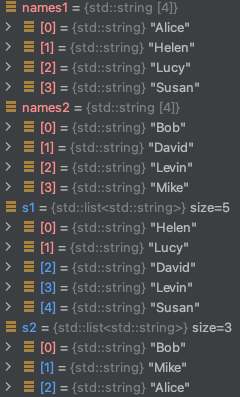####单向链表（forward_list）

• 单向链表每个结点只有指向下个结点的指针，没有简单的方法来获取一个结点的前驱；
• 未定义insert、emplace和erase操作，而定义了insertafter、emplaceafter和erase_after操作，其参数与list的insert、emplace和erase相同，但并不是插入或删除迭代器p1所指的元素，而是对p1所指元素之后的结点进行操作；
• 不支持size操作。

####数组（array）

• array是对内置数组的封装，提供了更安全，更方便的使用数组的方式
• array的对象的大小是固定的，定义时除了需要指定元素类型，还需要指定容器大小。
• 不能动态地改变容器大小

####顺序容器的比较

• STL所提供的顺序容器各有所长也各有所短，我们在编写程序时应当根据我们对容器所需要执行的操作来决定选择哪一种容器。
• 如果需要执行大量的随机访问操作，而且当扩展容器时只需要向容器尾部加入新的元素，就应当选择向量容器vector；
• 如果需要少量的随机访问操作，需要在容器两端插入或删除元素，则应当选择双端队列容器deque；
• 如果不需要对容器进行随机访问，但是需要在中间位置插入或者删除元素，就应当选择列表容器list或forward_list；
• 如果需要数组，array相对于内置数组类型而言，是一种更安全、更容易使用的数组类型。

###插入迭代器和适配器 ####插入迭代器

• 用于向容器头部、尾部或中间指定位置插入元素的迭代器
• 包括前插迭代器（frontinserter）、后插迭代器（backinsrter）和任意位置插入迭代器（inserter） 例如：
```list<int> s;
back_inserter iter(s);
*(iter++) = 5; //通过iter把5插入s末尾```

####适配器 以顺序容器为基础构建一些常用数据结构，是对顺序容器的封装

• 栈(stack)：最先压入的元素最后被弹出
• 队列(queue)：最先压入的元素最先被出
• 优先级队列(priority_queue)：最“大”的元素最先被弹出 ####栈和队列模板 （1）栈模板
`template <class T, class Sequence = deque<T> > class stack;`

（2）队列模板

`template <class T, class FrontInsertionSequence = deque<T> > class queue;`

• s1 op s2 op可以是==、!=、<、<=、>、>=之一，它会对两个容器适配器之间的元素按字典序进行比较
• s.size() 返回s的元素个数
• s.empty() 返回s是否为空
• s.push(t) 将元素t压入到s中
• s.pop() 将一个元素从s中弹出，对于栈来说，每次弹出的是最后被压入的元素，而对于队列，每次被弹出的是最先被压入的元素
• 不支持迭代器，因为它们不允许对任意元素进行访问

####栈和队列不同的操作 （1）栈的操作

• s.top() 返回栈顶元素的引用

（2）队列操作

• s.front() 获得队头元素的引用
• s.back() 获得队尾元素的引用

（3）举例：利用栈反向输出单词

```#include "iostream"
#include "string"
#include "stack"
using namespace std;

int main() {
stack<char> s;
string str;
cin >> str; //从键盘输入一个字符串
//将字符串的每个元素顺序压入栈中
for (string::iterator iter = str.begin(); iter != str.end(); ++iter)
s.push(*iter);
//将栈中的元素顺序弹出并输出
while (!s.empty()) {
cout << s.top();//读头元素
s.pop();//取头元素
}
cout << endl;
return 0;
}

cmake-build-debug/untitled
deltitnu/gubed-dliub-ekamc```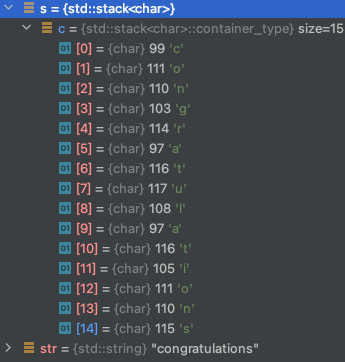####优先级队列

• 优先级队列也像栈和队列一样支持元素的压入和弹出，但元素弹出的顺序与元素的大小有关，每次弹出的总是容器中最“大”的一个元素。
`template <class T, class Sequence = vector<T> > class priority_queue;`
• 优先级队列的基础容器必须是支持随机访问的顺序容器。
• 支持栈和队列的size、empty、push、pop几个成员函数，用法与栈和队列相同。
• 优先级队列并不支持比较操作。
• 与栈类似，优先级队列提供一个top函数，可以获得下一个即将被弹出元素（即最“大”的元素）的引用。

##关联容器 特点： 每个关联容器都有一个键(key) 可以根据键高效地查找元素 ###分类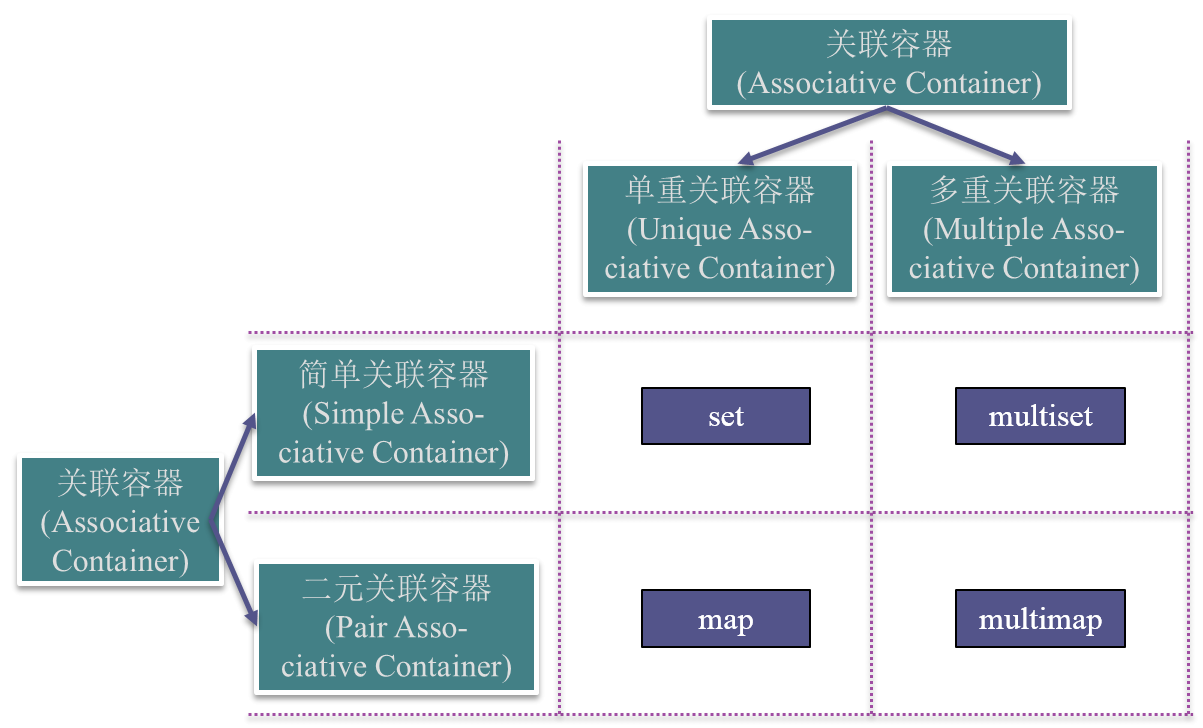（1）单重关联容器(set和map) 键值是唯一的，一个键值只能对应一个元素 （2）多重关联容器(multiset和multimap) 键值是不唯一的，一个键值可以对应多个元素 （3）简单关联容器(set和multiset)

• 容器只有一个类型参数，如set、multiset，表示键类型
• 容器的元素就是键本身

（4）二元关联容器(map和multimap)

• 容器有两个类型参数，如map、multimap，分别表示键和附加数据的类型
• 容器的元素类型是pair，即由键类型和元素类型复合而成的二元组

（5）无序关联容器

• C++11新标准中定义了4个无序关联容器：unorderedset、unorderedmap、unorderedmultiset、unorderedmultimap
• 不是使用比较运算符来组织元素的，而是通过一个哈希函数和键类型的==运算符。
• 提供了与有序容器相同的操作
• 可以直接定义关键字是内置类型的无序容器。
• 不能直接定义关键字类型为自定义类的无序容器，如果需要，必须提供我们自己的hash模板。

###接口

• 插入：insert
• 删除：erase
• 查找：find
• 定界：lowerbound、upperbound、equal_range
• 计数：count

###集合(set)

```#include <set>
#include <iterator>
#include <utility>
#include <iostream>
using namespace std;

int main() {
set<double> s;
while (true) {
double v;
cin >> v;
if (v == 0) break;  //输入0表示结束
//尝试将v插入
pair<set<double>::iterator,bool> r=s.insert(v);
if (!r.second)  //如果v已存在，输出提示信息
cout << v << " is duplicated" << endl;
}
//得到第一个元素的迭代器
set<double>::iterator iter1=s.begin();
//得到末尾的迭代器
set<double>::iterator iter2=s.end();
//得到最小和最大元素的中值
double medium=(*iter1 + *(--iter2)) / 2;
//输出小于或等于中值的元素
cout<< "<= medium: ";
copy(s.begin(), s.upper_bound(medium), ostream_iterator<double>(cout, " "));
cout << endl;
//输出大于或等于中值的元素
cout << ">= medium: ";
copy(s.lower_bound(medium), s.end(), ostream_iterator<double>(cout, " "));
cout << endl;
return 0;
}

1 2.5 5 3.5 5 7 9 2.5 0
5 is duplicated
2.5 is duplicated
<= medium: 1 2.5 3.5 5
>= medium: 5 7 9```

###映射（MAP）

• 映射与集合同属于单重关联容器，它们的主要区别在于，集合的元素类型是键本身，而映射的元素类型是由键和附加数据所构成的二元组。
• 在集合中按照键查找一个元素时，一般只是用来确定这个元素是否存在，而在映射中按照键查找一个元素时，除了能确定它的存在性外，还可以得到相应的附加数据。

```#include <iostream>
#include <map>
#include <utility>
using namespace std;

int main() {
map<string, int> courses;
//将课程信息插入courses映射中
courses.insert(make_pair("CSAPP", 3));
courses.insert(make_pair("C++", 2));
courses.insert(make_pair("CSARCH", 4));
courses.insert(make_pair("COMPILER", 4));
courses.insert(make_pair("OS", 5));
int n = 3;      //剩下的可选次数
int sum = 0;    //学分总和
while (n > 0) {
string name;
cin >> name;    //输入课程名称
map<string, int>::iterator iter = courses.find(name);//查找课程
if (iter == courses.end()) {    //判断是否找到
cout << name << " is not available" << endl;
} else {
sum += iter->second;    //累加学分
courses.erase(iter);    //将刚选过的课程从映射中删除
n--;
}
}
cout << "Total credit: " << sum << endl;    //输出总学分
return 0;
}

OS
COMPILER
C++
Total credit: 11```

```#include <iostream>
#include <map>
#include <cctype>
using namespace std;

int main() {
map<char, int> s;   //用来存储字母出现次数的映射
char c;     //存储输入字符
do {
cin >> c; //输入下一个字符
if (isalpha(c)){ //判断是否是字母：isalpha(c)若为英文字母，返回非0（小写字母为2，大写字母为1）。若不是字母，返回0。
c = tolower(c); //将字母转换为小写
s[c]++;      //将该字母的出现频率加1
}
} while (c != '.'); //碰到“.”则结束输入
//输出每个字母出现次数
for (map<char, int>::iterator iter = s.begin(); iter != s.end(); ++iter)
cout << iter->first << ": " << iter->second << "  ";
cout << endl;
return 0;
}

isalpha112a.
a: 3  h: 1  i: 1  l: 1  p: 1  s: 1```

####多重集合和多重映射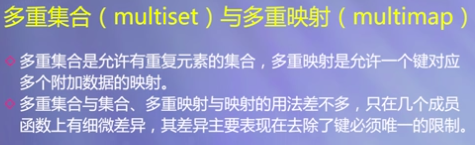##函数对象 ###函数对象

• 一个行为类似函数的对象
• 可以没有参数，也可以带有若干参数
• 其功能是获取一个值，或者改变操作的状态。 例： （1）普通函数就是函数对象 （2）重载了“()”运算符的类的实例是函数对象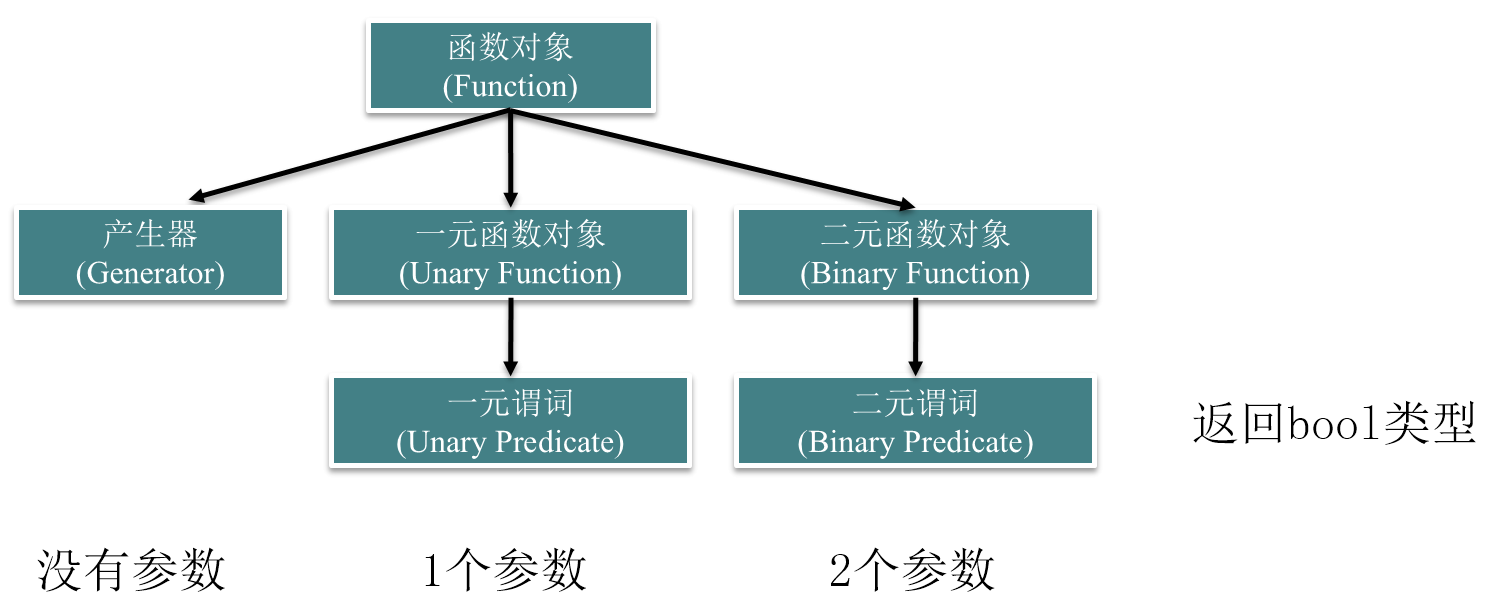```template<class InputIterator, class Type, class BinaryFunction>
Type accumulate(InputIterator first, InputIterator last, Type val, BinaryFunction binaryOp);```

（1）通过定义普通函数

```#include <iostream>
#include <numeric> //包含数值算法头文件
using namespace std;

//定义一个普通函数
int mult(int x, int y) { return x * y; };

int main() {
int a[] = { 1, 2, 3, 4, 5 };
const int N = sizeof(a) / sizeof(int);
cout << "The result by multipling all elements in a is "
<< accumulate(a, a + N, 1, mult)  //对a内的数据进行"运算"，binaryOp为用二元函数对象表示的某种"运算"，val为计算的初值
<< endl;
return 0;
}

（2）通过重载类的“()”运算符（例10-14）

```#include <iostream>
#include <numeric> //包含数值算法头文件
using namespace std;

class MultClass{  //定义MultClass类
public:
//重载操作符operator()
int operator() (int x, int y) const { return x * y; }
};
int main() {
int a[] = { 1, 2, 3, 4, 5 };
const int N = sizeof(a) / sizeof(int);
cout << "The result by multipling all elements in a is "
<< accumulate(a, a + N, 1, MultClass()) //将类multclass传递给通用算法
<< endl;
return 0;
}

####STL提供的函数对象 （1）用于算术运算的函数对象： 一元函数对象(一个参数) ：negate 二元函数对象(两个参数) ：plus、minus、multiplies、divides、modulus （2）用于关系运算、逻辑运算的函数对象(要求返回值为bool) 一元谓词(一个参数)：logical_not 二元谓词(两个参数)：equalto、notequalto、greater、less、greaterequal、lessequal、logicaland、logical_or

```#include <iostream>
#include <numeric>   //包含数值算法头文件
#include <functional>  //包含标准函数对象头文件
using namespace std;

int main() {
int a[] = { 1, 2, 3, 4, 5 };
const int N = sizeof(a) / sizeof(int);
cout << "The result by multipling all elements in A is "
<< accumulate(a, a + N, 1, multiplies<int>())//multiplies<int>()：二元函数对象(两个参数)
<< endl; //将标准函数对象传递给通用算法
return 0;
}```

```#include <functional>
#include<iostream>
#include<vector>
#include<algorithm>
using namespace std;

int main() {
int intArr[] = { 30, 90, 10, 40, 70, 50, 20, 80 };
const int N = sizeof(intArr) / sizeof(int);
vector<int> a(intArr, intArr + N);
cout << "before sorting:" << endl;
copy(a.begin(),a.end(),ostream_iterator<int>(cout,"\t"));//输出
cout << endl;

sort(a.begin(), a.end(), greater<int>());//重排序：greater<int>()逆序

cout << "after sorting:" << endl;
copy(a.begin(),a.end(),ostream_iterator<int>(cout,"\t"));//输出
cout << endl;

sort(a.begin(), a.end(), less<int>());//重排序：greater<int>()逆序

cout << "again sorting:" << endl;
copy(a.begin(),a.end(),ostream_iterator<int>(cout,"\t"));//输出
cout << endl;
return 0;
}

before sorting:
30	90	10	40	70	50	20	80
after sorting:
90	80	70	50	40	30	20	10
again sorting:
10	20	30	40	50	70	80	90```

###函数适配器 （1）绑定适配器：bind1st、bind2nd

• 将n元函数对象的指定参数绑定为一个常数，得到n-1元函数对象

（2）组合适配器：not1、not2

• 将指定谓词的结果取反

（3）函数指针适配器：ptr_fun

• 将一般函数指针转换为函数对象，使之能够作为其它函数适配器的输入。
• 在进行参数绑定或其他转换的时候，通常需要函数对象的类型信息，例如bind1st和bind2nd要求函数对象必须继承于binary_function类型。但如果传入的是函数指针形式的函数对象，则无法获得函数对象的类型信息。

（4）成员函数适配器：ptrfun、ptrfun_ref

• 对成员函数指针使用，把n元成员函数适配为n + 1元函数对象，该函数对象的第一个参数为调用该成员函数时的目的对象
• 也就是需要将“object->method()”转为“method(object)”形式。将“object->method(arg1)”转为二元函数“method(object, arg1)”。

####绑定适配器

• binder2nd的实例构造通常比较冗长，bind2nd函数用于辅助构造binder2nd，产生它的一个实例。
• binder1st和bind1st，将一个具体值绑定到二元函数的第一个参数。

```template<class InputIterator, class UnaryPredicate>
InputIterator find_if(InputIterator first, InputIterator last, UnaryPredicate pred);```

```#include <functional>
#include<iostream>
#include<vector>
#include<algorithm>
using namespace std;

int main() {
int intArr[] = { 30, 90, 10, 40, 70, 50, 20, 80 };
const int N = sizeof(intArr) / sizeof(int);
vector<int> a(intArr, intArr + N);
vector<int>::iterator p = find_if(a.begin(), a.end(), bind2nd(greater<int>(), 40));
if (p == a.end())
cout << "no element greater than 40" << endl;
else
cout << "first element greater than 40 is: " << *p << endl;
return 0;
}```

####组合适配器

• 对于一般的逻辑运算，有时可能还需要对结果求一次逻辑反。
• unarynegate和binarynegate实现了这一适配功能。STL还提供了not1和not2辅助生成相应的函数对象实例，分别用于一元谓词和二元谓词的逻辑取反。

```#include <functional>
#include<iostream>
#include<vector>
#include<algorithm>
using namespace std;

bool g(int x, int y) {
return x > y;
}

int main() {
int intArr[] = { 30, 90, 10, 40, 70, 50, 20, 80 };
const int N = sizeof(intArr) / sizeof(int);
vector<int> a(intArr, intArr + N);
vector<int>::iterator p;
p = find_if(a.begin(), a.end(), bind2nd(ptr_fun(g), 40));//找到第一个大于40的数：90
if (p == a.end())
cout << "no element greater than 40" << endl;
else
cout << "first element greater than 40 is: " << *p << endl;
p = find_if(a.begin(), a.end(), not1(bind2nd(greater<int>(), 15)));//not1逻辑（greater）取反，即比12小的
if (p == a.end())
cout << "no element is not greater than 15" << endl;
else
cout << "first element that is not greater than 15 is: " << *p << endl;

p = find_if(a.begin(), a.end(), bind2nd(not2(less<int>()), 15));
if (p == a.end())
cout << "no element is  greater than 15" << endl;
else
cout << "first element that is  greater than 15 is: " << *p << endl;
return 0;
}

first element greater than 40 is: 90
first element that is not greater than 15 is: 10
first element that is  greater than 15 is: 30```

```#include <functional>
#include <iostream>
#include <vector>
#include <algorithm>
using namespace std;

struct Car {
int id;
Car(int id) { this->id = id; }
void display() const { cout << "car " << id << endl; }
};

int main() {
vector<Car *> pcars;
vector<Car> cars;
for (int i = 0; i < 5; i++)
pcars.push_back(new Car(i));
for (int i = 5; i < 10; i++)
cars.push_back(Car(i));
cout << "elements in pcars: " << endl;
for_each(pcars.begin(), pcars.end(), std::mem_fun(&Car::display));//将成员函数适配（mem_fun）为普通函数对象
cout << endl;

cout << "elements in cars: " << endl;
for_each(cars.begin(), cars.end(), std::mem_fun_ref(&Car::display));//mem_fun_ref的参数是对象的引用；mem_fun的参数是对象的指针
cout << endl;

for (size_t i = 0; i < pcars.size(); ++i)
delete pcars[i];

return 0;
}

elements in pcars:
car 0
car 1
car 2
car 3
car 4

elements in cars:
car 5
car 6
car 7
car 8
car 9```

##算法 ###算法特点

• STL算法本身是一种函数模版
• 通过迭代器获得输入数据
• 通过函数对象对数据进行处理
• 通过迭代器将结果输出
• STL算法是通用的，独立于具体的数据类型、容器类型 ###算法分类 ####不可变序列算法
• 不直接修改所操作的容器内容的算法
• 用于查找指定元素、比较两个序列是否相等、对元素进行计数等

```template<class InputIterator, class UnaryPredicate>
InputIterator find_if(InputIterator first, InputIterator last, UnaryPredicate pred);```

####可变序列算法

• 可以修改它们所操作的容器对象
• 包括对序列进行复制、删除、替换、倒序、旋转、交换、分割、去重、填充、洗牌的算法及生成一个序列的算法

```template<class ForwardIterator, class T>
InputIterator find_if(ForwardIterator first, ForwardIterator last, const T& x);```

• 对序列进行排序
• 对两有序序列进行合并
• 对有序序列进行搜索
• 有序序列的集合操作
• 堆算法

```template <class RandomAccessIterator , class UnaryPredicate>
void sort(RandomAccessIterator first, RandomAccessIterator last, UnaryPredicate comp);```

• 求序列中元素的“和”、部分“和”、相邻元素的“差”或两序列的内积
• 求“和”的“+”、求“差”的“-”以及求内积的“+”和“·”都可由函数对象指定

```template<class InputIterator, class OutputIterator, class BinaryFunction>
OutputIterator partial_sum(InputIterator first, InputIterator last, OutputIterator result, BinaryFunction op);```

##习题 ###STL （1）定义 const int b ={9,2,3};调用insertionSort函数， insertionSort(b,3),可以执行成功。 正确。 分析：const类型不是 Sortable概念的模型 （2）set::insert(const value_type＆val); 函数的返回值类型是什么?

• iterator
• 指针
• bool
• pair＜iterator,bool＞（对） （3）```#include <deque>
#include <iostream>
using namespace std;

/*
* 队列：3  1  4  8  6
*   (出)头          尾(进)
*/
int main()
{
deque<int> iDeque;
iDeque.push_back(3);//队列尾部插入元素：push_back()
iDeque.push_back(1);
iDeque.push_back(4);
iDeque.push_back(8);
iDeque.push_back(6);
while (!iDeque.empty()) {
cout << "取出元素" << iDeque.front() << endl;//队列头部查看第一个元素：front()
iDeque.pop_front();//队列头部弹出第一个元素：pop_front()
}
return 0;
}

（4）```#include <iostream>
#include <algorithm> //程序中使用了算法find和sort
#include <functional> //程序中使用了函数对象greater
using namespace std;

int main()
{
int a = {3, 2, 5, 6, 4, 1, 9, 8};
cout << "元素9的索引是：" << find(a, a+8, 9) - a << endl;//find返回的是地址
cout << "升序排序：" << endl;
sort(a, a+8);//默认是升序
for (int i = 0; i < 8; i ++)
cout << a[i] << ' ';
cout << endl;
cout << "升序排序：" << endl;
sort(a, a+8, less<int>());//使用函数对象实现降序排序
for (int i = 0; i < 8; i ++)
cout << a[i] << ' ';
cout << endl;
cout << "降序排序：" << endl;
sort(a, a+8, greater<int>());//使用函数对象实现降序排序
for (int i = 0; i < 8; i ++)
cout << a[i] << ' ';
cout << endl;
return 0;
}

1 2 3 4 5 6 8 9

1 2 3 4 5 6 8 9

9 8 6 5 4 3 2 1```